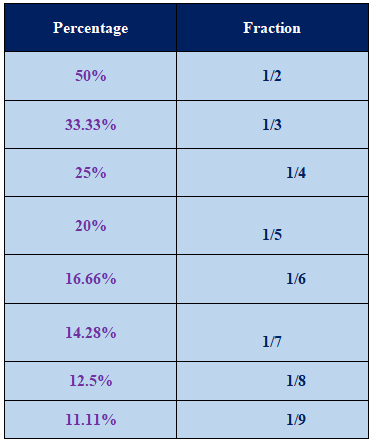# Percentage Formula

In mathematics, we most of the time come across with the problems related to percentage calculation and students mostly afraid of this things and they leave the problems in exam also.

In this article, we will see how we can find the percentage of any number within a second without taking any load. We can also find the decimal percentage easily.

We weekly go in market for the shopping of groceries, shoes, cloths and many things there will be some offers which are referred in the form of percentage that time we have to calculate orally the percentage values. Also, while in online shopping also there are lot of offers and we without calculating the percentage by seeing offer only we order that material.

So it is very important to know how percentage can be calculated easily and orally. Everyone should have to do the calculation to save money and be safe from blacking money.

### Percentage Formula:

Percentage is an important and effective chapter of mathematics which students have to learn in their curriculum of secondary education. Students often feel afraid to solve critical problems of percentage and leave them during exams.             In this process, they left behind their learning and become unable to cope with higher studies in mathematics. Students will learn about tactics of solving percentage problems from this article how to solve them with much load. They will get formulas of solving decimal percentage too. We can see application and need of percentage in every sphere of our daily life. Like when we go to market or shopping malls for buying groceries, shows, clothes, electronic gadgets and everything of our needs we see lots of offers in terms of percentage. At that time we have to calculate all less price of percentage orally. For that we should have complete knowledge about calculating percentage properly.

We see lots of offers in percentage on various products in online shopping and we often end up in ordering materials without calculating price. So, we must know how to calculate percentage orally within less period of time. Percentage is the value of number calculated in the fraction of 100, calculated over 100 and denoted as %. Percentage is just a number, it does not have any dimension and unit to express. Percentage can be both increase and decrease as per the problems. to solve problems of percentage we have to divide the number first with 100 and them multiply the resultant number by 100. Problems are often asked to convert percentage in decimal numbers. We can see percentage problem maximum related to marks obtained in examination. We have to calculate percentage by dividing obtained marks with total number then the resultant number multiplied by 100. Students should learn the specific formula to calculate decimal forms of percentage for solving problems correctly.

• In mathematics, percentage is the value of number or the ratio expressed as the fraction of 100.
• Percentage is calculated over 100 always and it is denoted by %.
• It has no dimensions and no unit as it is only a number.

In our markmemo percentage are mentioned, have you checked it?

For example:

You got total marks 650 out of 800 then what percentage you got?

• So this can be calculated as, obtained marks/ total marks * 100 = 650/ 800*100 = 81.25%
• Hence, the percentage you got would be 81.25%.

In general,

% = value/ total value *100

Example 1:

If you have to find 20% of 1200.

100% of 1200 = 1200

10% of 1200 = 120

1% of 1200 = 12

• Thus, from this we conclude that when we have to find the percentage in tens digit then we have to shift unit digit after decimal point.
• And for calculating percentage in units digit we have shift the last two digits after the decimal point.

Hence, 10% of 1200 = 120.0

And 1% of 1200 = 12.00

Similarly, 2% of 1200 = 1% + 1% = 12 + 12 = 24

5 % of 1200 = 5*1% of 1200 = 5*12 = 60

25% of 1200 = 20% + 5 % = 2*10% + 5*1%

= 2*120 + 5*12

= 240 + 60

25% of 1200 = 300

Again, 12.5% of 1200 =?

Then, 12.5% of 1200 = 12% + 0.5%

= 12*1% of 1200 + 0.5% of 1200

= 12*12 + 6

= 144 + 6 = 150

12.5% of 1200 = 150 = half of 25%

Or by using formula we can find it as,

Let x be the 25% of 1200 then we write as,

x/ 1200 * 100 = 25%

x = (25*1200) / 100 = 25* 12 = 25*10 + 25*2

x = 250 + 50 = 300

Hence, 300 will be the 25% of 1200.

In this way we can easily find the percentage in fraction also.

There lots of methods to find the percentage, but we have to choose only one so that we will get the perfection in that method only.

Example 2:

If you have to find 24.5% of 1200 then,

24.5% of 1200 = 25% of 1200 – 0.5% of 1200

= 300 – ½ of 1%

= 300 – ½*12

= 300 – 6= 294

24.5% of 1200 = 294

Or by directly we can find 24.5% of 1200 as follows

24.5% of 1200 = 24.5*1% of 1200

= 24.5 * 12

24.5% of 1200 = 294

Example 3:

98.5% of 1200 = 98.5*1% of 1200 = 98.5 * 12 = 1182

Or

98.5% of 1200 = 100% of 1200 – 1.5% of 1200

= 1200 – 1% – 0.5%

= 1200 – 12 – 6 = 1200 – 18

98% of 1200 = 1182

The following table shows the percentage in terms of fraction.Updated: May 4, 2023 — 12:54 pm